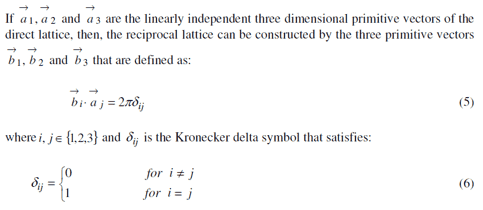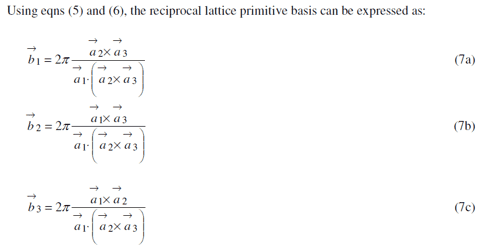# Reciprocal lattice basis

• I
sam45
TL;DR Summary
I am trying to understand how to determine the reciprocal lattice basis from the lattice basis vectors
may someone explain to me or show me the steps of how equations 7a, 7b, 7c were determined from equations 5 and 6Gold Member
You can check directly that those formulas for the ##b_i## satisfy the equations that you want. For example, ##b_1\cdot a_1=2\pi\frac{a_1\cdot (a_2\times a_3)}{a_1\cdot (a_2\times a_3)}=2\pi##.

Since the vectors ##a_i## are linearly independent, the equations ##b_i\cdot a_j=2\pi\delta_{ij}## can be uniquely solved for the ##b_i##, and hence the above are the unique solutions.

If you wanted to figure out these formulas from scratch, you could argue like this: since ##b_1## is orthogonal to both ##a_2## and ##a_3##, you know that ##b_1=c_1 (a_2\times a_3)## for some constant ##c_1##. Then the equation ##a_1\cdot b_1=2\pi## let's you solve for ##c_1##, etc.

sam45
hello,

thank you. yes, but my question is how are they solved for bi i.e. what are the steps to find b1 for example

thank you

•